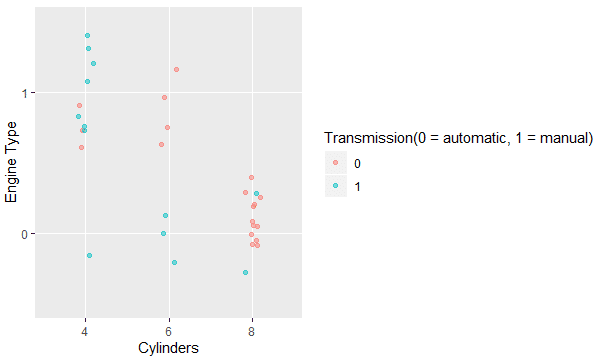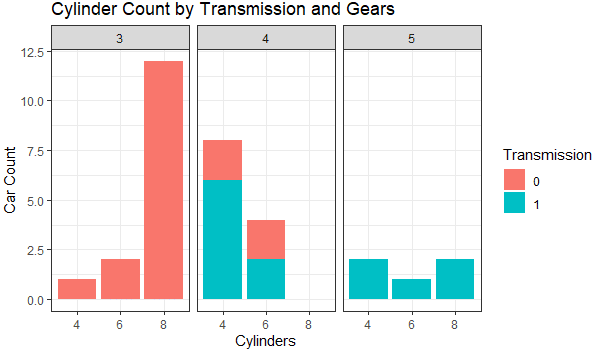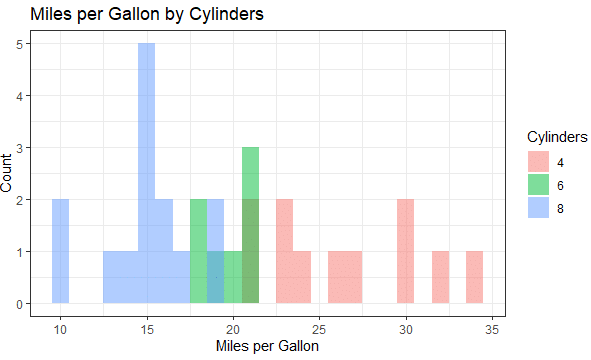## Data Visualization

Data visualization is a technique used for the graphical representation of data. By using elements like scatter plots, charts, graphs, histograms, maps, etc., we make our data more understandable. Data visualization makes it easy to recognize patterns, trends, and exceptions in our data. It enables us to convey information and results in a quick and visual way.
It is easier for a human brain to understand and retain information when it is represented in a pictorial form. Therefore, Data Visualization helps us interpret data quickly, examine different variables to see their effects on the patterns, and derive insights from our data.

Want to get certified in R! Learn R from top R experts and excel in your career with Intellipaat’s R Programming certification!

In the era of Big Data, data visualization has become vital in analyzing and interpreting huge amounts of data and in making wise and data-driven decisions.

###R programming provides comprehensive sets of tools such as in-built functions and a wide range of packages to perform data analysis, represent data and build visualizations.
Data visualization in R can be performed in the following ways:

• Base Graphics
• Grid Graphics
• Lattice Graphics
• ggplot2

Now that we have grasped what data manipulation in R programming is, and different ways to perform it, let’s have a look at the summary of the topics that will be discussed in this tutorial:

• Data Visualization in R
• Base R Graphics
• Data Visualization with ggplot2 package

Check out the top R Programming Interview Questions to learn what is expected from R professionals!

### Base R Graphics

R provides some built-in functions which are included in the graphics package for data visualization in R.
In this tutorial, we are going to use the default mtcars dataset for data visualization in R.

```#To load graphics package
library("graphics")
library("datasets")
data(mtcars)
#To analyze the structure of the dataset
str(mtcars)```

Output:

```'data.frame':  32 obs. of  11 variables:
\$ mpg : num  21 21 22.8 21.4 18.7 18.1 14.3 24.4 22.8 19.2 ...
\$ cyl : num  6 6 4 6 8 6 8 4 4 6 ...
\$ disp: num  160 160 108 258 360 ...
\$ hp  : num  110 110 93 110 175 105 245 62 95 123 ...
\$ drat: num  3.9 3.9 3.85 3.08 3.15 2.76 3.21 3.69 3.92 3.92 ...
\$ wt  : num  2.62 2.88 2.32 3.21 3.44 ...
\$ qsec: num  16.5 17 18.6 19.4 17 ...
\$ vs  : num  0 0 1 1 0 1 0 1 1 1 ...
\$ am  : num  1 1 1 0 0 0 0 0 0 0 ...
\$ gear: num  4 4 4 3 3 3 3 4 4 4 ...
\$ carb: num  4 4 1 1 2 1 4 2 2 4 ...```

It contains data about the design, performance and fuel economy of 32 automobiles from 1973 to 1974, extracted from the 1974 Motor Trend US magazine.

#### The plot() Function

The plot() function is used to plot R objects.
The basic syntax for the plot() function is given below:

`plot(x,y,type,main,sub,xlab,ylab,asp,col,..)`

x:–  The x coordinate of the plot, a single plotting structure, a function, or an R object
y:– The Y coordinate points in the plot (optional if x coordinate is a single structure)
type:– ‘p’ for points, ‘l’ for lines, ‘b’ for both, ‘h’ for high-density vertical lines, etc.
main:– Title of the plot
sub:– Subtitle of the plot
xlab:– Title for the x-axis
ylab:– Title for the y-axis
asp :- Aspect ratio(y/x)
col:– Color of the plot(points, lines, etc.)
For example:

```#To plot mpg(Miles per Gallon) vs Number of cars
plot(mtcars\$mpg, xlab = "Number of cars", ylab = "Miles per Gallon", col = "red")```

Output:Here, we get a scatter/dot plot wherein we can observe that there are only six cars with miles per gallon (mpg) more than 25.

```#To find relation between hp (Horse Power) and mpg (Miles per Gallon)
plot(mtcars\$hp,mtcars\$mpg, xlab = "HorsePower", ylab = "Miles per Gallon", type = "h", col = "blue")```

Output:Here, we can observe that hp and mpg have a negative correlation, which means that as Horse Power increases Miles per Gallon decreases.

#### Barplot

It is used to represent data in the form of rectangular bars, both in vertical and horizontal ways, and the length of the bar is proportional to the value of the variable.
For example:

```#To draw a barplot of hp
#Horizontal
barplot(mtcars\$hp,xlab = "HorsePower", col = "cyan", horiz = TRUE)
#Vertical
barplot(mtcars\$hp, ylab = "HorsePower", col = "cyan", horiz = FALSE)```

Output:Histogram
It is used to divide values into groups of continuous ranges measured against the frequency range of the variable.
For example:

```#To find histogram for mpg (Miles per Gallon)
hist(mtcars\$mpg,xlab = "Miles Per Gallon", main = "Histogram for MPG", col = "yellow")```

Output:Here, we can observe that there are six cars with MPG between 10 and 15.

Become a Data Scientist with this complete Data Science Training Course!

#### Boxplot

It is used to represent descriptive statistics of each variable in a dataset. It represents the minimum, first quartile, median, third quartile, and the maximum values of a variable.

```#To draw boxplots for disp (Displacement) and hp (Horse Power)
boxplot(mtcars[,3:4])```

Output:

### Data Visualization in R with ggplot2 package

The ggplot2 package in R is based on the grammar of graphics, which is a set of rules for describing and building graphs. By breaking up graphs into semantic components such as scales and layers, ggplot2 implements the grammar of graphics.
The ggplot2 grammar of graphics is composed of the following:

• Data
• Layers
• Scales
• Coordinates
• Faceting
• Themes

ggplot2 is one of the most sophisticated packages in R for data visualization, and it helps create the most elegant and versatile print-quality plots with minimal adjustments. It is very simple to create single- and multivariable graphs with the help of the ggplot2 package.

###The three basic components to build a ggplot are as follows:

• Data:– Dataset to be plotted
• Aesthetics:- Mapping of data to visualization
• Geometry/Layers:- Visual elements used for the data

The basic syntax for ggplot is given below:

`ggplot(data = NULL, mapping = aes()) + geom_function()`
```#To Install and load the ggplot2 package
install.packages(“ggplot2”)
library(ggplot2)```

In this tutorial, we are going to use the mtcars dataset from the datasets package in R that can be loaded as follows:

```#To load datasets package
library("datasets")
data(mtcars)
#To analyze the structure of the dataset
str(mtcars)```

#### Scatter Plots

To draw a scatter plot of cyl(Number of Cylinders) and vs(Engine Type(0 = V-shaped, 1 = straight)), run the code below:

```#Since the following columns have discrete(categorical) set of values, So we can
convert them to factors for optimal plotting
mtcars\$am <- as.factor(mtcars\$am)
mtcars\$cyl <- as.factor(mtcars\$cyl)
mtcars\$vs <- as.factor(mtcars\$vs)
mtcars\$gear <- as.factor(mtcars\$gear)
#To draw scatter plot
ggplot(mtcars, aes(x= cyl , y= vs)) + geom_point()```

Output:Since this plot has a lot of overlapped values, which is known as overplotting, we will use geom_jitter() function to add a certain amount of noise to avoid it.

```#Here width argument is used to set the amount of jitter
ggplot(mtcars, aes(x= cyl , y= vs)) + geom_jitter(width = 0.1)```

Output:Here, we can also use the argument alpha to set the transparency of the points to further reduce overplotting for data visualization in R.

```#Transparency set to 50%
ggplot(mtcars, aes(x= cyl , y= vs)) + geom_jitter(width = 0.1, alpha = 0.5)```

Output:With ggplot2, we can plot multivariate plots effectively.
For example:
To draw a scatter plot of cyl(Number of Cylinders) and vs(Engine Type(0 = V-shaped, 1 = straight)) according to am Transmission (0 = automatic, 1 = manual), run the following code:-

```#We use the color aesthetic to introduce third variable with a legend on the right side
ggplot(mtcars, aes(x= cyl,y= vs,color = am)) + geom_jitter(width = 0.1, alpha = 0.5)```
```#To add the labels
ggplot(mtcars, aes(x= cyl , y= vs ,color = am)) +
geom_jitter(width = 0.1, alpha = 0.5) +
labs(x = "Cylinders",y = "Engine Type", color = "Transmission(0 = automatic, 1 = manual)")```

Output:

```#To plot with shape =1 and size = 4
ggplot(mtcars, aes(x = wt, y = mpg, col = cyl)) +
geom_point(size = 4, shape = 1, alpha = 0.6) +
labs(x = "Weight",y = "Miles per Gallon", color = "Cylinders")```

Output:

#### Bar Plots

```#To draw a bar plot of cyl(Number of Cylinders) according to the Transmission type
using geom_bar() and fill()
ggplot(mtcars, aes(x = cyl, fill = am)) +
geom_bar() +
labs(x = "Cylinders", y = "Car count", fill = "Transmission")```
```#To find the proprtion, we use position argument,as follows:
ggplot(mtcars, aes(x = cyl, fill = am)) +
geom_bar(position = "fill") +
labs(x = "Cylinders",y = "Proportion",fill = "Transmission")```

Output:

#### Themes

It is used to change the attributes of non-data elements of our plot like text, lines, background, etc. We use the theme_function() to make changes to these elements for data visualization in R.
Some of the commonly used theme function is as follows:

• theme_bw() :- For white background and gray grid lines
• theme_gray:- For gray background and white grid lines
• theme_linedraw:- For black lines around the plot
• theme_light:- For light gray lines and axis
• theme_void:- An empty theme, useful for plots with non-standard coordinates or for drawings
• theme_dark():- A dark background designed to make colors pop out

For example:

```ggplot(mtcars, aes(x = cyl, fill = am)) +
geom_bar(position = "fill") +
theme_classic()+
labs(x = "Cylinders",y = "Proportion",fill = "Transmission")```

Output:

#### Faceting

It is used to further drill down data and split the data by one or more variables, and then plot the subsets of the data altogether for optimum data visualization in R.
For example:

```#To facet the following plot according to gear(Number of Gears(3,4,5)), we use
facet_grid() function as follows:
ggplot(mtcars, aes(x = cyl, fill = am)) +
geom_bar() +
facet_grid(.~gear)+
#facet_grid(rows ~ columns)  theme_bw() +  labs(title = "Cylinder count by transmission and Gears",x = "Cylinders",       y = "Count",fill = "Transmission")```

Output:

##Go through this R Programming Course in London to get a clear understanding of R Programming!

#### Histograms

```#To plot a histogram for mpg (Miles per Gallon),
according to cyl(Number of Cylinders), we use the geom_histogram() functiong
gplot(mtcars, aes(mpg,fill = cyl)) +
geom_histogram(binwidth = 1)+
theme_bw()+
labs(title = "Miles per Gallon by Cylinders",x = "Miles per Gallon",y =
"Count",fill = "Cylinders")```
```#To show overlapping, we set position to identity and alpha to 0.5
ggplot(mtcars, aes(mpg,fill = cyl)) +
geom_histogram(binwidth = 1,position = "identity", alpha = 0.5)+
theme_bw()+
labs(title = "Miles per Gallon by Cylinders",x = "Miles per Gallon",y = "Count",fill = "Cylinders")```

Output:

```#To overcome overlapping, we can use the frequency polygon, as follows:
ggplot(mtcars, aes(mpg, color = cyl)) + geom_freqpoly(binwidth = 1)+
theme_bw()+
labs(title = "Miles per Gallon by Cylinders",x = "Miles per Gallon",y = "Count",fill = "Cylinders")```

Output:

#### Boxplot

```#To draw a Box plot
ggplot(mtcars, aes(x = cyl,y = mpg)) +
geom_boxplot(fill = "cyan", alpha = 0.5) +
theme_bw() +
labs(title = "Cylinder count vs Miles per Gallon",x = "Cylinders",
y = "Miles per Gallon")```
```#To draw a Box plot
ggplot(mtcars, aes(x = cyl,y = mpg,fill = am)) +
geom_boxplot( alpha = 0.5) +
theme_bw() +
labs(title = "Cylinder vs MPG by Transmission",x = "Cylinders",
y = "Miles per Gallon",fill = "Transmission")```

Output:In this tutorial, we have learned what data visualization in R is and various techniques for data visualization in R including Base R Graphics, and the ggplot2 package.

Have a look at this informative blog on why should you learn R Programming to clarify all your doubts!